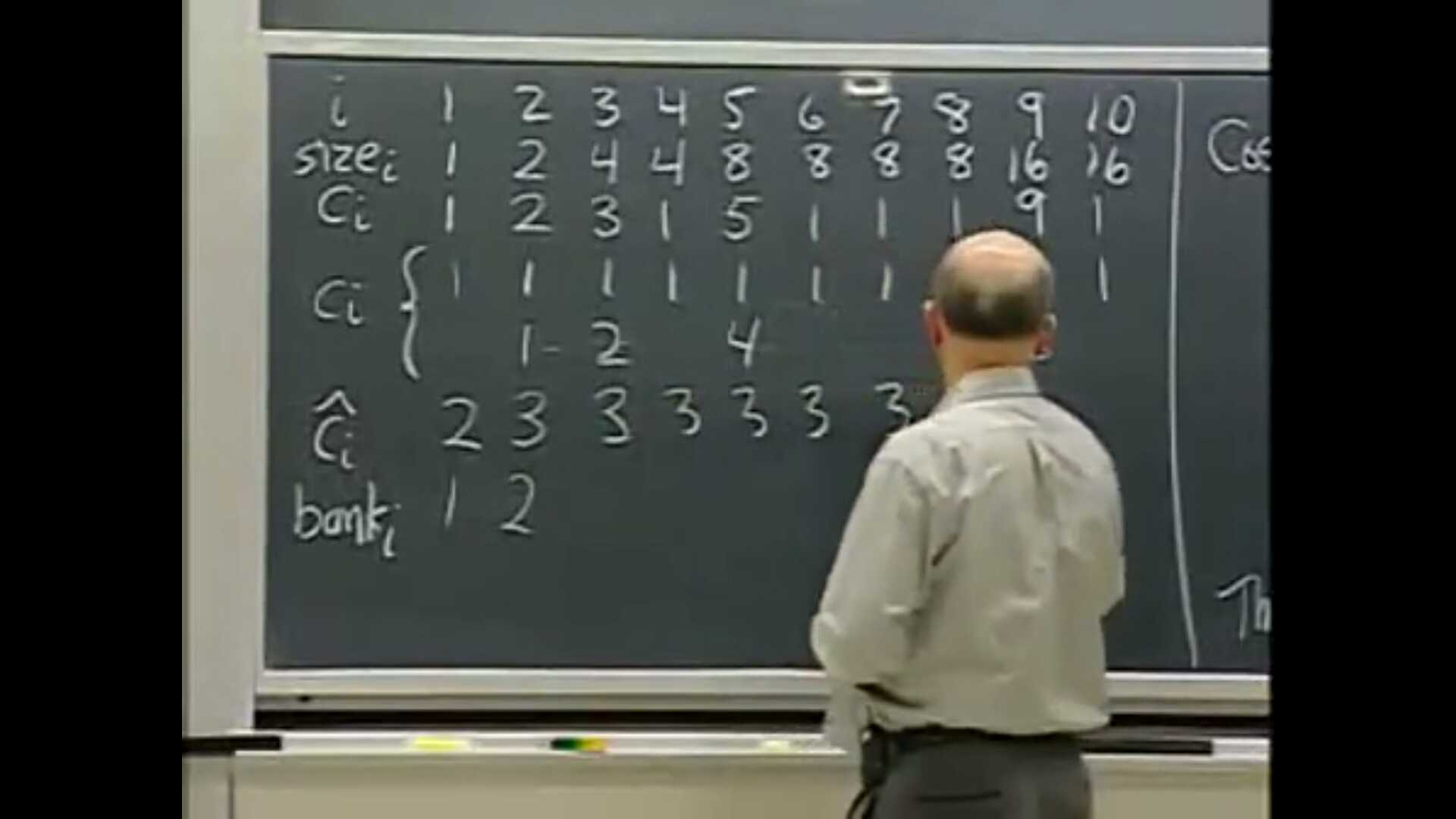# KMP

KMP算法是一种前缀搜索方法，也就是说在搜索窗口内从前向后逐个读入文本字符，搜索窗口中文本和模式串的最长公共前缀。相对于前缀搜索，还有后缀搜索（Boyer-Moore、Horspool、Sunday）、子串搜索等方法。

## KMP对朴素算法的优化

• 朴素的字符串匹配算法复杂度为O(n*m)，对于长n的文本串S，从位置[0..(n-m)]开始搜索匹配长m的模式串，对于每个位置至多需要m次比较。假设在(i,j)位置发生失配，那么接下来应当从(i-j+1,0)位置开始重新匹配。
• 容易发现其中有一些比较和回退操作是多余的。例如，假定模式串为P'ABCDABD'，文本串为S='ABC ABCDAB'，假设从(0,0)位置开始匹配，在(3,3)位置发现' ''D'不等。此时朴素算法直接在(1,0)位置重新开始匹配，但实际上在刚才比较过程中可以得到(0..3,0)位置都是不行的，因为'B''C'' '都不等于'A'，因此可以从(4,0)重新开始匹配。
• 另一个例子，考虑新的S='ABCDAB ABCDABCDABDE'，在(6,6)处发现' ''D'不等失配。同时发现P[0..1]P[4..5]都能匹配S[4..5]（当然S[4..5]S[0..1]都能匹配P[0..1]'AB'，但目前我们考虑只移动模式串），由此可以尝试从(4,2)（注意j变成了2不是0）开始重新匹配，容易发现S并没有发生回退，而P右移了4位，用自己P[0..1]而不是P[4..5]'AB'去匹配S[4..5]了。
• 因此可以得到KMP算法的思想：如果模式串P(i,j)处失配文本串S，那么可以知道至少P[0..(j-1)]S[i..(i+j-1)]是匹配的，既然如此，不妨可以利用已经匹配的这段长度，只回退（将串向右移动）模式串P，不回退文本串S（注意在KMP算法过程中对文本串S的操作只有递增一个）。因此通过KMP算法，假设在(i,j)位置发生失配，那么接下来应当右移符号串j-f(j)长度，从(i,f(j))位置开始重新匹配（注意此时失配前P[0..(j-1)]S[i-(j-1)..i-1]已经匹配的部分会和P[f(j)-(j-1)..f(j)-1]重新匹配），其中f为next函数，将在下节中详细介绍；特别地，当j = 0时，我们应当从(i+1,0)开始匹配。可以得到这样算法的复杂度为O(n+m)

## KMP算法和next函数

• next函数f(j)指出了查找下一个匹配时的模式串（这里可以理解为P[0..m-1]的子串P[0..j]）的回退距离（模式串P向右移动的距离），或者可以被称作最长边界。用形式方法来描述即，对于模式串P[0..j]来说，f(j) = max(k)，这里k满足P[(j-1)-(k-1)..(j-1)]P[(j-k）..(j-1)] = P[0..(k-1)]（注意这里是j-1k-1，在别的定义/实现方式中可能使用j和k）。
这里要求是最大的k也就是最长边界，原因是考虑到可能存在多个k，为了不丢失匹配，回退距离应当尽可能小。
• 特别地，当j = 0时，即对于(i,0)位置的失配，指定f(0) = -1，这意味着我们应当递增文本串S的指针i，从下一位置匹配。
• 为了计算所有的f(1..m)，可以根据定义进行计算，朴素的方法需要O(m^2)的复杂度。但是可以采用dp实现线性复杂度的算法：
f(j) = k，即已知最大的k满足P[j-k...j-1] = P[0...k-1]，现在求f(j+1)
• 如果P[k] = P[j]，则f(j+1) = k+1
• 如果P[k]!=P[j]，于是显然P[0..k]显然不是P[0..j]的后缀（注意根据f定义下标分别是到jk），所以只能在P[0..(k-1)]上寻找P[0..j]的后缀，但是P[0..(k-1)]也不一定就满足条件，当然可以对P[0..(k-1)]的所有前缀判O(k^2)次，但是也有简单办法，那就是利用失配前已经匹配了的结果。请在这里停顿并尝试自己推一下下面的过程，并体会其中的奇妙之处。
k= k2 = f(k)，继续寻找，如果此时P[k2]=P[j]，那就符合了，否则还要继续寻找。为什么说只要比较P[k2]=P[j]就行了呢？前面的k-1的长度不需要比较了么？因为k2是最大的满足P[k-k2..k-1] = P[0..k2-1]（也就是P[0..k2-1]的最长边界长度），而注意到我们发现P[k]!=P[j]，说明至少P[0..k-1]P[j-k..j-1]是匹配的，因此P[0..k2-1] = P[k-k2..k-1] = P[j-k..j-1]，即我们新找到的P[0..k2-1]也和P[j-k..j-1]匹配。
• 下面对P='ABCDABD'构造next函数。根据上文，首先有f(0)=-1。接下来计算f(1)，也就是在(i,j = 1)发生失配。我们需要找到此时P[0..(j-1)]='A'的某个后缀同时也是P的前缀，显然并不存在。

## 求next函数和KMP算法比较

KMP算法实际上是求模式串P[0..m]对文本串S[0..n]长度为m+1的边界（如果存在即找到）。
next函数实际上是求模式串的某个前缀P[0..j]P[0..j]长度最大的边界。

## 相关资料

KMP算法有很多教程，每个教程都给出了自己的一套理解方法，没有一个我能够完全看懂。在学习过程中，我参照了Wikipedia的解释，其中适当后缀(proper suffix)，指的是不是自己本身的后缀，也就是“真后缀”。

## 复杂度分析

### 摊还分析

1. 聚合法
聚合法就是求出所有操作加起来最坏情况的总代价，然后除以总操作数。对于std::vector的添加元素操作来说，假设初始大小是1，倍增因子是m，要添加$n$个元素，需要重新分配内存$\lceil log_{m}{n} \rceil$次，第$i$次分配内存需要移动$m^{i-1}$个元素，总共需要$\sum_{i=1}^{\lceil log_{m}{n} \rceil}{m^{i-1}} = O(n)$次移动操作，另需要push_back $n$次。因此复杂度为O(1)

2. 核方法
MIT的算法导论课公开课老师讲这个的时候在黑板上写了2 3 3 3…对这个记忆犹新核方法的原理就是把复杂度低的操作承担一部分复杂度高的操作的代价，从而证明算法复杂度的上界。定义$c_i$为第$i$个操作的真实代价，定义$\hat{c}_i$为摊还代价。在第$i$时刻，定义信用为$\sum_{i=1}^n{\hat{c}_i} - \sum_{i=1}^n{c_i}$，选取的摊还代价要保证信用始终非负，因为一旦信用是负数，那么这个操作实际上是在开销上界内不能完成的
上图是一个倍增因子m为2的动态表std::vector，$size_i$表示添加第$i$项时的表达大小，真实代价$c_i$由两部分组成，一部分是添加进表的固定成本（$c_i$第一行），另一部分是把元素复制到新表中的成本（$c_i$第二行），$bank_i$就是信用。对于$m=2$的情况，可以发现$\hat{c}_i$取3即可，这包括自己的真实代价，移动表的前一半的代价，和移动表的后一半的代价。对于普遍的倍增因子m，后面m-1的元素要m个元素的移动代价，加上添加进表的1，摊还代价取$1 + \frac{m}{m - 1}$，特别地$i = 1$时只要支付自己的移动代价，故摊还代价为$1$，于是等式左边$\sum_{2}^{n}{(\frac{m}{m - 1})} + n = \frac{2nm - n - m}{m - 1}$，等式右边$n + \sum_{0}^{\lceil log_{m}{n} - 1 \rceil}{m^i} = n + \frac{n - 1}{m - 1} = \frac{nm - 1}{m - 1}$，发现左边恒大于右边。

3. 势能法
势能法和核方法基本上是相同的，区别在于核方法要先假设摊还代价，而势能法先考虑的信用和（也就是势）。对于有的题目势能法比核方法简单。对于上面的$m=2$的动态表，定义势函数$\phi(D_i) = 2i - 2^{\lceil log_{2}{i} \rceil}$，由于$i = 2^{log_{2}{i} }$，所以可以得到式子恒为正。因此满足势函数的基本要求$\phi(D_0) = 0$和$\phi(D_i) \ge 0$。下面计算摊还代价$\hat{c}_i = c_i + \phi(D_i) - \phi(D_{i - 1})$，其中$c_i = \begin{cases} i \, , \, (i - 1) & ((i - 1) - 1) == 0 \\1 \, , \, otherwise \end{cases}$。通过对$i$的讨论，可以发现每个操作的摊还代价是3，这和前面的核方法形成了印证。
下面考虑倍增因子为m的情况，定义势函数为$\phi(D_i) = 2i - m^{\lceil log_{m}{i} \rceil}$，可以进行类似的计算，得到每个操作的摊还代价是$1 + \frac{m}{m - 1}$

# Trie

Trie（/ˈtraɪ/，字典树，前缀树）将多个字符串组成一棵有序树。一个节点的所有子孙都有相同的前缀，也就是这个节点对应的字符串，而根节点对应空字符串。在构造Trie树时，需要用flag数组标记每个节点是否可能是某个字符串的结尾，而不能仅靠“无路可走”来断定，例如对于单词表[b, bb]bbb都是合法的单词。

## 例题

HDU 1247是Trie的简单应用，首先把每个字符串添加到Trie树上，然后对于每个字符串，搜索他的每个前缀，对于任意前缀，如果它产生的字符串属于这个单词表（碰到flag为true的节点），那么紧接着继续往下搜索看能不能搜到第二个字符串。这道题注意要搜索每个前缀，考虑下面的样例

ha
hat
word
hatword


# AC自动机

AC自动机（Aho-Corasick automation）能够匹配多个字符串，可以用来在线性时间内解答字符串S中出现了哪些字典D中的模式串的问题。从算法思想上来看，AC自动机结合了Trie和KMP的思路，虽然它的出现甚至要比KMP早。对我来说AC自动机的思想显得比KMP“自然”点，我们在Trie树上走，如果走不下去（失配）了，我们就回退到某个上层的节点。在这点上和KMP很相近，因为KMP也不回退文本串。